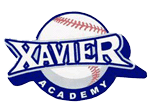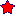TuesdaySep 29/207:10 pmMST .teamname { text-shadow: 3px 3px 1px #002799; font-family: 'Alfa Slab One', cursive; font-size: 45px; font-weight: bold; font-style: italic; color: #AAAAAA; zoom: 1; filter: progid:DXImageTransform.Microsoft.DropShadow(OffX=3, OffY=3, Color=#002799) progid:DXImageTransform.Microsoft.Blur(pixelradius=1); } FX BASEBALL ACADEMYROSTER www.fxbaseball.com

Attendance:

Edmonton
AB

Players
 Name POS B T Nicholas Allen OF R R Michael Anderson P/1st R R Ryan Anderson RHP R R Connor Baldry RHP/INF R R Andrew Banford R R Marshall Becker IF R R Adam Beller RHP/1B R R Matthew Berner OF/RHP L R Cole Bezle-Johnson C R R Brandon Billesberger IF L R Craig Bitensky RHP/IF R R Chris Blowers OF/RHP R R Carson Bokenfohr 1B/3B L R Matthew Bondarchuk OF/RHP L R Drew Boyer OF/P R R Jakob Brinkhof IF/OF R R Devon Briscoe C/INF L R Mitchell Brodie LHP/OF R L Dylaney Brown SS/OF/RHP R R Braeden Burns C R R Taylor Burns P/3rd R R Landon Bymoen RHP R R Jackson Campbell R L Connor Cassidy OF R R Hayden Chies P/OF R R Dylan Chudek R R Shane Clackson RHP/OF R R Taylor Collins RHP/IF/OF R R Thomas Copeland RHP/IF R R Matthew Corbiere OF R R Alex Cordingly 3B R L Matthew Courneya RHP/OF R R Erika Couterille-Arcand R R Jonas Coyes 1B/LHP L L Derrick Dahlside LHP/OF L R Brendan Dawidowski C R R Stephen Daynard IF/RHP L R Kade Denney C/INF R R Austin Doris C/INF R R Austen Doroshuk OF/RHP R R Jonathan Down 2B/SS L R Finnigan Duffield LHP/OF L L Jacob Duffin C/INF L R Tyler Dunsmore OF/P/Util R R Louis Dwyer OF R R Ben Erwin RHP/INF R R Chris Ewanik C L R Dirk Eymundson C/IF/RHP L R Josh Fisher RHP/INF R R Riley Fisher IF L R Zaine Foth-Thomas LHP/1B R L Mason French RHP/OF R R Chase Gallagher OF R R Lucas Galloway C/utility R R Justin Ganser OF/P R R Scott Gillespie OF/RHP R R Connor Girard RHP/OF R R Bradley Goodwin IF R R Andrew Grafton IF/P R R Tyler Grayston RHP/IF R R Jarvis Greiner P/SS R R Andrew Grieder OF/LHP R L Daniel Grimard RHP/OF R R J.J Grimm P/IF R R Tom Grimm P/IF L R Luis Grisanti INF/RHP R R Luis Gristani OF/1B R R Jacob Hamelin INF R R Joshua Hamelin 1B R R William Hanna R R Chase Harris IF/RHP R R Lee Hendrick 3B/RHP L R Kohl Herman LHP/OF L L Tsar Hester LHP/OF R L Paige Hildebrandt RHP/INF R R Brandon Hill INF/RHP R R Tyler Hill OF/RH L R Kyle Hockley OF/RHP R R Brett Holden 1B/C L R Greg Hollman RHP/INF R R Tanner Horbasenko R R Zach Horman 1B/LHP L L Aidan Huggins SS/RHP R R Josh Hunter OF R R Braxton Hyland C R R Spencer Imeson OF L R Nolan James INF R R Taylor Jones INF/RHP R R Tanner Karpinski 2B/3B R R Joseph Kemper-Vela INF R R Wesley Kenneth RHP/IF R R Marlee Kereliuk IF R R Ethan Kerr OF R R Bret Kirkpatrick C/RHP R R Ryan Koop R R Brendan Kordyback 1B/SS L L Andrew Kotscherofski IF/RHP R R Keegan Kwong C/RHP R R Evan Lacoursiere LHP/INF L R Tauren Langley INF/RHP R R Eric LeBlanc OF R R Cameron Leflar IF R R Stephane Legault IF/C/RHP L R Ryan Lehman OF/INF/RHP R R Nemanja Lilic RHP/OF R R Ty Locicero SS/C R R Aidan Lucas RHP/IF R R Nicole Luchanski 2nd R R Roger Lukasik IF/P R R Aaron Lystang C S R Aaron Macdonald C R R Richard Mallett OF/INF/RHP R R Reid Marchichiw IF/RHP R R Ashton Martin IF/OF R R Isaiah Martin RHP/IF R R Brock Mason 1B/LHP L L Thurman Matthews OF/LHP L L Cassie Mattuli IF L L Brandon McCaffrey OF R R Hunter McDonald IF/RHP R R Matt McGee IF/RHP R R Tehgan McGillis OF R R Ben McLeod IF/RHP R R Dexter McLeod IF/RHP R R Bo Meiklejohn 1B/LHP L L Jacob Melville IF/RHP R R Mina Mesic OF R R Sarah Michaud C R R Jordan Moger R R Hayley Morin INF R R Tatum Morin INF/RHP R R Riley Moskaluk RHP/OF R R Jesse Murowchuk RHP/IF R R Levi Muth C/IF/RHP R R Chris Mycyk OF/IF L R Tetsuhei Nakashima-Ochoa OF/LHP L L Jordan Nault OF R R Chase Neely OF/RHP R R Koalson Nykiforuk IF/OF L R Kienan O'Connor 3B/RHP L R Connor O'Hara OF/LHP R L Evan Olsen OF R R Anthony Olson IF/RHP R R Dean Olson OF/RHP R R Gage Otto OF/IF R R Travis Ouellet 1B/RHP L R Ethan Pacholok OF R R Saige Pacholok OF/P R R Joe Pasychnyk RHP/3B L R Garrett Perlette RHP/IF R R Trevor Petty RHP/IF R R Katelyn Phillips-Derkacz INF R R Ian Pollard OF/RHP/3B R L Jesse Poniewozik RHP/OF R R Gage Popadynetz OF/RHP R R Alexandra Poznikoff OF R R Kyle Prather OF/LHP L L Kyle Radke IF/C R R Steven Rea INF/RHP L R Darrin Reay R R Matthew Reiniger INF L R Colten Repka OF/P R R Cameron Rich RHP/OF R R David Richards IF/OF RHP L R Sean Robbins C/IF R R Luke Roberts RHP/OF R R Julius Robinson INf/RHP R R Russell Rockwell RHP/OF L R Ryley Ross IF R R Andrew Roth IF/RHP R R Michael Rowland-Kalynchuk RHP/INF R R Matt Rude 2nd/P R R Erik Sabrowski LHP/1B R L Steven Savard OF R R Cole Schneider IF/RHP R R Burke Seifrit IF/P R R Brett Semeniuk RHP/IF L R Jaret Semeniuk IF/C/OF L R Matthew Senkoe-Gough INF/RHP R R Derek Shedden IF/RHP S R Codey Shrider IF/OF/RHP R R Matthew Shurvell OF/1B R R Sam Siddall OF/RHP R R Kyle Slattery OF/P R R Nick Smiley C/RHP S R Lee Spicer OF/IF R R Katryna Springer C R R Vasilios Stamatakis INF R R Matthew Stebner 1B/LHP L L Ryan Thrasher RHP/C/2B R R Jacob Tompalski 2B R L Gavin Turinek IF/OF/RHP R R Jackson Vandermeer IF/OF R R Hunter Ward R R Nicolas Ward-Ogima INF R R Logan Wedgewood C/RHP R R Nathan Wedgewood 3B/RHP/C R R Max Welz OF R R Chance Wheatley IF L R Dakota Wheatley R L Darnell Whittaker OF/RHP R R Joshua Williams RHP/OF S R Michael Williams LHP/OF R L Ned Winter RHP/OF R R Brenden Wiun RHP/C/INF L R Nicholas Yekimchuk OF L R Tate Yuzda C/OF L R Zach Yuzda INF/RHP L R

Coaches
 Name POS 2nd POS Rob Boik Florida Trip Coach Mark Randall Academy Coordinator Pitching Coordinator Sheen Bromley Lead Instructor Hitting Coordinator Nate Hay Strength and Conditioning Coordinator Athletic Therapist Ethan Pacholok Strength and Conditioning Coach Chris James Fall and Florida Coach Carlos Duncan Fall Baseball Coach Matt McGee Fall Baseball CoachLineup Cards, Dugout Charts, Pitching Charts, Free Team Pages, Free League Pages